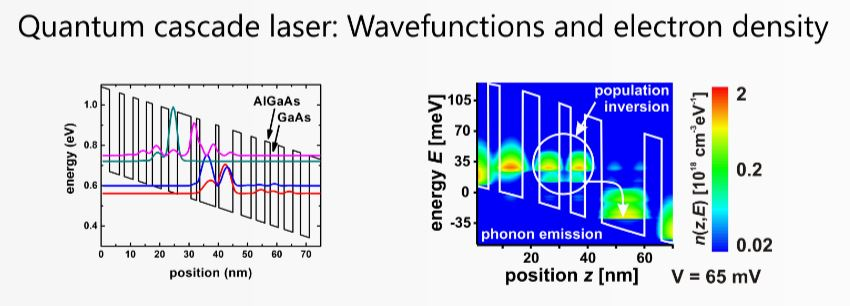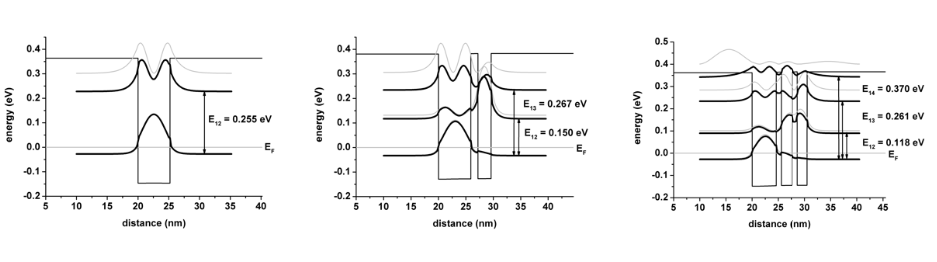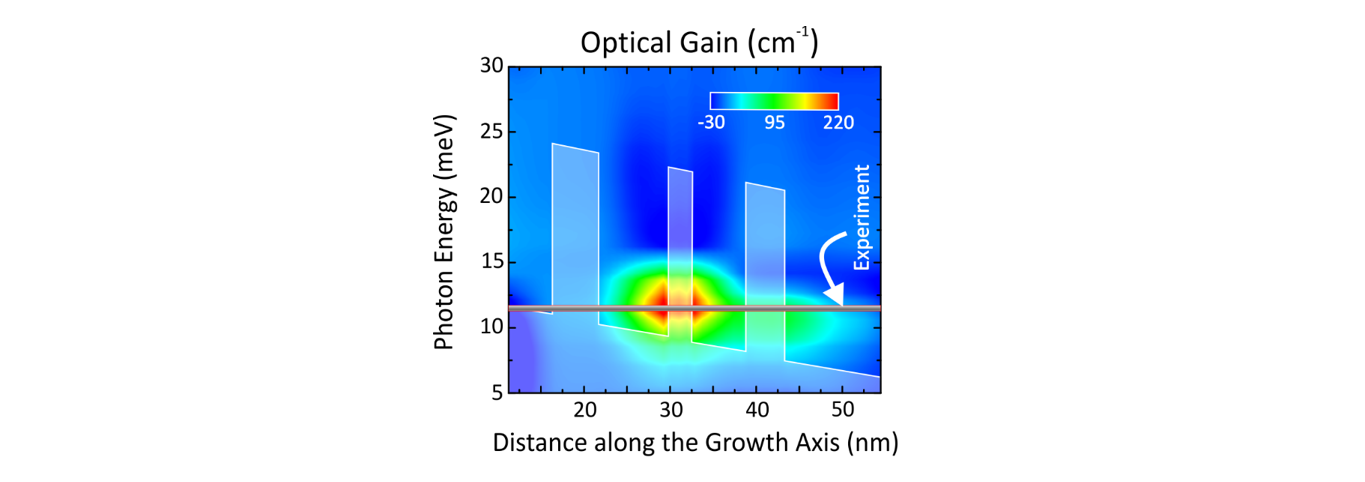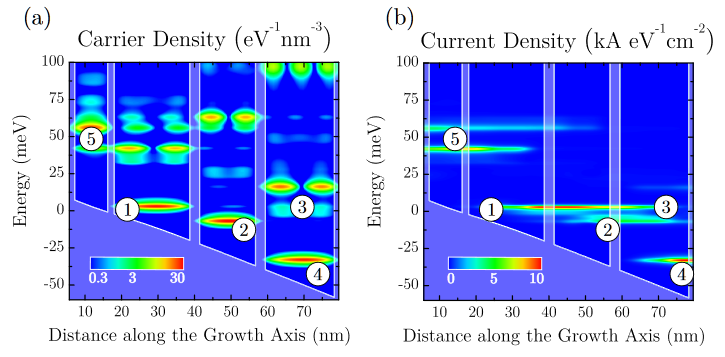# Quantum cascade lasers

A quantum cascade laser is a very unique semiconductor laser that has been first demonstrated at Bell Laboratories in 1994.

## Quantum cascade laserWikipedia has some nice information on QCLs: Quantum cascade laser

nextnano.NEGF

• Nonequilibrium Green's function (NEGF) method for calculating I-V curves and gain
• NEGF quantum transport based on the code written by Thomas Grange
• Documentation
• Download nextnano.NEGF including an evaluation license for a few months.

## Video

This video (mpg4, 23 MB) shows how a QCL works. The first 10 seconds summarize the features of our software, the remaining 20 seconds show the animated simulation results. At 20 seconds, the QCL starts to lase: The electron density (figure on the right) shows the population inversion, i.e. the higher state has a larger electron population than the lower lasing state.

## Leaflet on QCL simulations

Information on nextnano software for QCLs

## Presentation

A good introduction into the nextnano.NEGF software is the tutorial talk Modeling electron transport in quantum cascade lasers given by Thomas Grange at the International Quantum Cascade Laser School and Workshop (Cassis/France, 2018).

## Tutorials

If you are interested in further tutorials, please contact stefan.birner [at] nextnano.com.

## InGaAs multiple quantum wellWhat you can learn:
• single-band effective mass vs. 8-band k·p, InGaAs/AlInAs
• self-consistent Schrödinger-Poisson equation, 8-band k·p Schrödinger equation

## ResultsThis figure shows the calculated electron density in a THz QCL.This figure shows the calculated gain of a THz QCL.

## Featuresnextnano software for quantum transport

1) nextnano.NEGF

• Nonequilibrium Green's function (NEGF) method for calculating I-V curves and gain
• NEGF quantum transport based on the code written by Thomas Grange
• Documentation

nextnano.MSB

• Nonequilibrium Green's function (NEGF) method for calculating I-V curves and gain based on the multi-scattering Büttiker probe model (MSB)
• NEGF quantum transport based on the MSB method (code written by Peter Greck)
• nextnano.MSB

2) nextnano3 & nextnano++

• self-consistent Schrödinger and Poisson equations
• 8x8 k.p Schrödinger equation
• strain
• Documentation (nextnano3)
• Documentation (nextnano++)

## Publications

The following two papers give a very basic introduction to QCL modeling.

Our recent publications on QCL modeling: